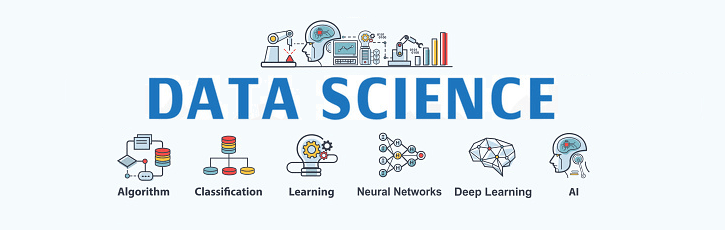DS Tutorial

# Machine Learning Algorithms | Python

Machine learning algorithms play a pivotal role in tackling intricate questions that surpass the capacity of manual analysis. Their purpose lies in learning from data, enabling models to autonomously improve and enhance performance through experience, with minimal human intervention. Diverse machine learning algorithms employ distinctive analytical approaches, harnessing data-driven insights to unravel complex patterns and phenomena, ultimately yielding valuable solutions to a wide array of challenging problems.So, they can be divided into 3 broad categories:

1. Supervised Learning
2. Unsupervised Learning
3. Reinforcement Learning

## Supervised Learning

In the domain of Supervised Learning algorithms, the input dataset is segregated into separate training and test datasets. During the training process, a model is constructed by making predictions and subsequently correcting errors. This iterative procedure refines the model's performance. Supervised learning encompasses the majority of machine learning algorithms, with two prominent subcategories: Regression, which deals with predicting continuous numeric values, and Classification, which focuses on predicting categorical labels or classes for data points.

Regression algorithms
1. Simple Linear Regression
2. Multiple Linear Regression
3. Ordinary Least Squares Regression
4. Polynomial Regression
Classification algorithms
1. Logistic Regression
2. K-Nearest Neighbor
3. Decision Tree
4. Random Forest
5. Support Vector Machine

## Unsupervised Learning

In Unsupervised Learning algorithms, there is no target or outcome variable provided for prediction or estimation. Instead, the algorithms autonomously learn intrinsic features from the given dataset. When encountering new data, the algorithms employ the learned features to determine the data's inherent characteristics or patterns. Unsupervised Learning finds applications in tasks like clustering, where it groups similar data points together, and feature reduction, where it reduces the dimensionality of the data to capture essential information efficiently.

## Reinforcement Learning

Reinforcement Learning revolves around making decisions to maximize rewards in a given situation. It is a feedback-driven technique where an agent learns to navigate an environment by taking actions and observing the consequences of those actions. Unlike supervised learning, Reinforcement Learning operates without labeled data and relies on automatic learning through feedback mechanisms to optimize the agent's behavior over time.For machine learning beginners eager to explore the fundamentals of this technique, here is a quick tour of the top Machine Learning algorithms commonly utilized by data scientists.